## General Mechanical

•Gmtassi
Subscriber

Hello everybody,

I will try to explain briefly what is the problem I am facing. Basically, I have a model made up of 32 bodies and 4 different materials that can be used. I have to find which combination body-material guarantee the minimum compliance once fixed a target weight. It is a sort of topology optimization problem.

I found this code that I am trying to use that seems could help me:

```!###############################################################################################################################
! MATSWAP.MAC
! Created by Joe Woodward at PADT,Inc.
! Created on 2/12/2016
!
! Usage: Create Named Selections, Holder1, Holder2, etc.,for BODIES using the materials that you want to use.
! Create Named Selections called MatSwap1, MatSwap2, etc. for the groups of BODIES for which you want to swap materials.
! Set ARG1 equal to the Holder number that has the material to give to MatSwap1.
! Set ARG2 equal to the Holder number that has the material to give to MatSwap2.
! And so on....
! A value of 0 will not swap materials for that given group.
!
! Use as is. No Modification to this command snippet is necessary.
!###############################################################################################################################
/prep7
*CREATE,MATSWAP,MAC
*if,arg1,NE,0,then
*get,isthere,COMP,holder%arg1%,TYPE
*get,swapgood,COMP,matswap%ARG2%,TYPE
*if,isthere,eq,2,then
esel,s,,,holder%arg1%
*get,newmat,elem,ELNEXT(0),ATTR,MAT
!swap material for Body 1
*if,swapgood,eq,2,then
esel,s,,,matswap%ARG2%
emodif,all,mat,newmat
*else
/COM,The Named Selection - MatSwap%ARG2% is not set to one or more bodies
*endif
*else
/COM,The Named Selection Holder%ARG1% is not set to one or more bodies
*endif
*endif
*END
MATSWAP,ARG1,1 !Use material from Holder1 for Swap1
MATSWAP,ARG2,2 !Use material from Holder1 for Swap2
MATSWAP,ARG3,3 !Use material from Holder1 for Swap3
MATSWAP,ARG4,4 !Use material from Holder1 for Swap4
MATSWAP,ARG5,5 !Use material from Holder1 for Swap5
MATSWAP,ARG6,6 !Use material from Holder1 for Swap6
MATSWAP,ARG7,7 !Use material from Holder1 for Swap7
MATSWAP,ARG8,8 !Use material from Holder1 for Swap8
MATSWAP,ARG9,9 !Use material from Holder1 for Swap9

alls
/solu```

This code swap the material contained in a body "Holder" to the material Swap1,2,3 which number is the number of the ARG (input of the commands).

THE PROBLEM IS: the number of arguments is limited to 9. There is a way that I can increase this number? If I use this command twice how can I modify this code in order to take the Swap10,11,12... during the second time the code is run?

I wish I was clear...

Thank you in advance to anyone who will help me!

•peteroznewman
Subscriber

If I understand you, there is an analysis that will compute the compliance and weight for each body/material combination and you have 4x32 = 128 analyses to compute, then you can sort the analyses by compliance result and select the winning combination by finding the one closest to the target weight that has the minimum compliance.

Are there a few parameters that characterize all four materials, such as density and Young's Modulus?  If so, you can have just one material and a parameter study that has four rows containing the values that represent each of the four materials.

Similarly, are there a few parameters that characterize all 32 body shapes? If so, you can have just one model and one material and a parameter study that has 128 rows.

Regards,

Peter

•Gmtassi
Subscriber

Thank you Peter!

you have completely understood the problem. I found very interesting what you said. So, the materials that I am going to use are the representation in Ansys of 4 different types of infills for an object that will be 3D printed. Considering those infills isotropic (just as a first approximation) and using just one material, I will have 4 different densities and for each density a Young modulus. Is there a way to correlate the value of the density with that one of the Young modulus? In other words, at each density value correspond one and only young modulus. How can I set this constraint in Ansys?

Thank you again,

Gian.

•peteroznewman
Subscriber

Gian,

The relationship between Young's modulus and the density selected for the infill on a 3D printed part is not simple. That is because there is a solid fill skin on the part and only the interior has lower density. I would be curious to see the shapes of the bodies.

I have a 3D printer at work so I see the infill being used, but there is no way for me to get the geometry of the infill matrix out of the printer software. I am looking to purchase the optional license for STL and infill for SpaceClaim. That way I can have some actual infill matrix geometry, then I can simulate a three-point bending test and calculate the Flexural Modulus for that infill design.  Do you have access to the actual infill matrix geometry?  If you do, then that is how you would determine the Young's modulus, assuming bending is the key deformation.  If the part is just in tension, then simulate a tensile test and obtain the Young's modulus from that test.

Below, I show the automation available in Workbench to get results from four materials, assuming you have determined the Young's Modulus for each density.

In Engineering Data, you check the Parameter box as shown in the last column below for density and Young's Modulus.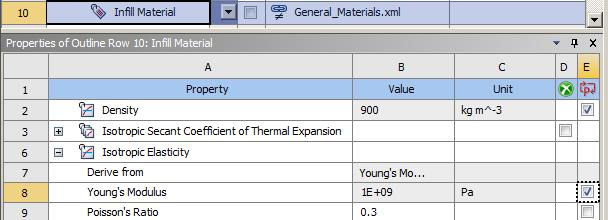Then in the model, select the body and click a P on the Mass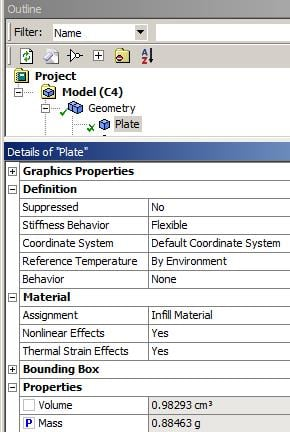Then on the results, click a P on the Maximum Deformation.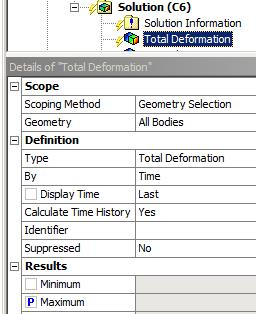Then in Workbench, open the Parameter Set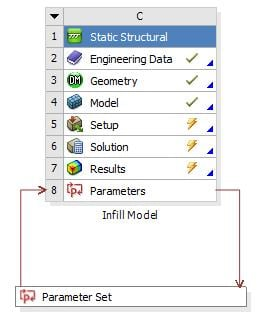An type in the four rows for Density and Young's Modulus.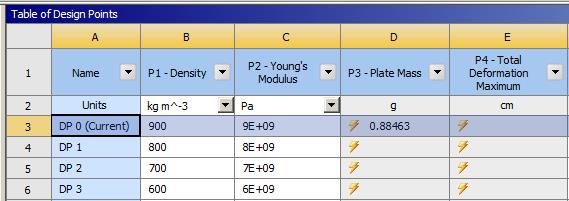Then you click Update All Design Points and the solver will run four times and fill out the results.

If you can also parameterize the body shape, you can add more columns and end up with 128 rows.

Regards,

Peter

•Gmtassi
Subscriber

Wow, thank you very much for your help.

It was really useful and I am going to use something similar I think, but I have another question. The infill will not be the same for each body. There will be (potentially) 4 different pattern inside the object that can be repeated in separate bodies. In other words, I have an object with 33 bodies: 32 "internal" bodies for which I am free to choose the infill pattern, while the other body, which is the external shape of the object and the internal "walls" that divide the internal bodies, is set to be completely solid.

So, if I well understood, I should do what you showed me above for every body, right? and actually I should make every possible combination of internal patterns. It seems impossible to do, due to the huge amount of simulation that are required, but I heard something about Design Optimization which use parameters (like those you showed me above) to optimize certain quantity. Do you think this will solve the problem? or in general, do you find any solution to that?

thank you again Peter,

Best regards,

Gian

•peteroznewman
Subscriber

Gian,

If you could show a picture of the internal bodies and the external shell, maybe a cross section view to see where the infill will go, that would help to visualize other approaches to your optimization problem.

You can't try all 4 materials in 32 bodies since that would be 1.8E18 analyses!

There is a method called Topology Optimization, where a large block of geometry is the initial design, then through iteration, low stress elements are deleted until only elements that are needed to carry the load are left. You might try that approach with your 32 bodies. Start with all 32 bodies solid filled, and let the Topology Optimizer hollow them out for you. At the end of the process, you don't need any infill, the volume element is either solid or air.

Best regards,

Peter

•Gmtassi
Subscriber

I already used Topology optimization package, and obtained avarage densities in the bodies and set a type of infill. So, I obtained a result. It is acceptable, but my concern was: this result is obtained from a reinterpretation of the analysis done by ansys. So I don't think it is a robust demonstration that this is the best solution. For this reason I thought about creating the 4 infill first and then let ansys to decide which one is the best for each body. in this way the output of ansys would be directly the solution of the problem.

I am not able now to show you the object cause i am not in the lab, but imagine a big cube divided in smaller cube by walls. each cube delimited by the walls is a body.

thanks again,

Gian

•peteroznewman
Subscriber

That's interesting that you started with Topology optimization and now are trying to further optimize the solution.

I have not done much with the ANSYS Design Optimization but I will share the following observations based on this 8 body model plus a wall. Each body has its own material designation cube1, cube1 2, cube1 3, etc. Each material has a Young's Modulus and density parameter so there are a total of 16 parameters.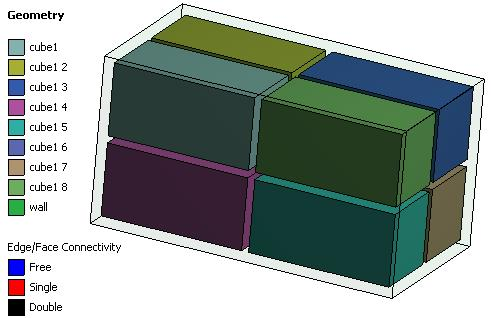This Static Structural Model had output parameters of Max Total Deformation and the mass of the 9 bodies. I haven't created a new variable that adds together the 9 masses, but I expect that is straightforward. I added to this parameter set the Response Surface model and the RS Optimization under that, as well as the Direct Optimization.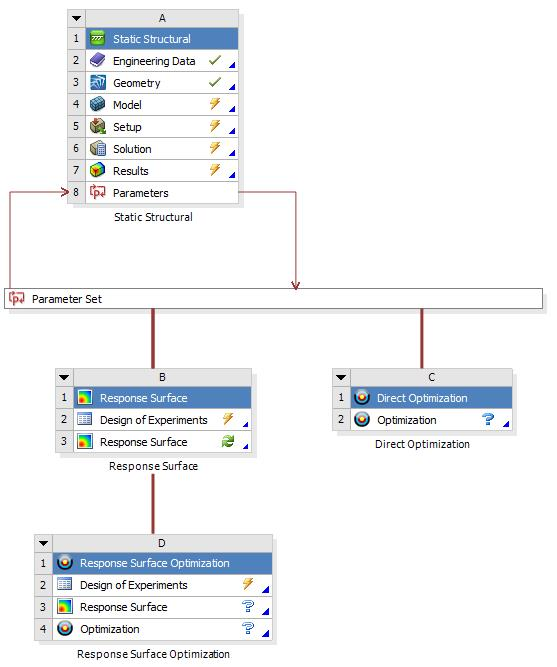It is possible to set each input parameter to four levels using Manufacturable Values.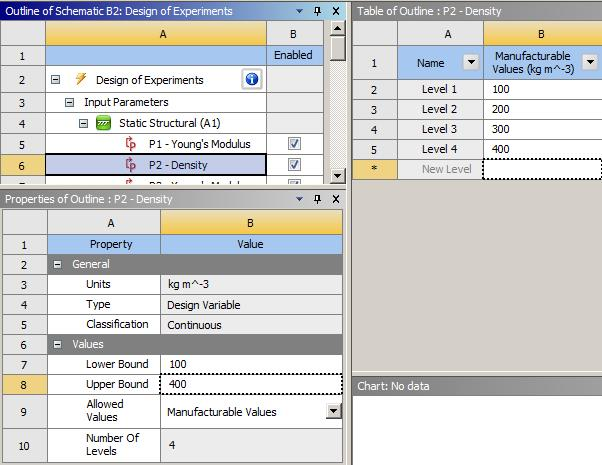Before I changed this one density to 4 levels, it was a continuous variable with an upper and lower limit. Part of the Response Surface is to create a DOE to cover the design space. For these 16 input parameter (2 material properties x 8 bodies), the DOE contained 289 rows that a Static Structural solution would be required to generate results for Max Deformation and total mass.

After those results are computed, you move to the Optimization, which uses various algorithms to minimize deformation subject to a target mass constraint but searching on the Response Surface, and not solving any more Static Structural models. That way, if the optimizer needs to take 1000 steps to find the optimum, it doesn't take long.

The Direct Optimization uses those same algorithms, but solves a Static Structural model for each search step. If the algorithm converges in less than 289 steps, then this is faster than the Response Surface optimization, otherwise it is slower.

Best regards,

Peter

•Gmtassi
Subscriber

Good morning Peter,

the fact that Young modulus is related to density like E=f(d)*E0 (where E0 is the Young modulus for the solid material, d is the relative density and f(d) a function that depend on the density and the geometry of the infill) could help to reduce the number of experiments? In other words, is there a way to write E in the material properties as a function of the density? I think in this way we halve the number of experiments required. Moreover, do you know how to set to minimize compliance as an objective?

In addition (this part is more an idea, and I am just asking your opinion), changing a little bit the point of view, how does Ansys work during topology optimization? It optimizes compliance changing relative density elements. If we believe it uses iteration as design of experiments, that would take infinite time due to the number of elements in a model. Instead I think it use a sort of sensibility analysis. If it does so, It would mean that ansys is able to compute the  derivative of the compliance (or strain energy)... So do you think it is possible to exploit this capability of ansys to solve my analysis quickly? I don't know if I was very clear in my idea. This idea come to me thinking that: How is it possible that, with so many variables in topology optimization (huge number of elements in a mesh and all the possible relative densities between 0 and 1) is it capable of solving this problem quickly while in my problem of just 32 variable (the density) it needs so many iteration/experiments?

I wish you can help me with those 2 doubts... Thanks, and I wish you a very nice weekend!

Best regards,

Gian

•peteroznewman
Subscriber

Good evening Gian,

I understand exactly what you mean, that there are only 32 variables in your case, each variable takes one of four values of density, and then there is an equation that calculates Young’s modulus from density.

You have to do the work to figure out what the equation is, but unfortunately, I don’t know how to encode that equation in Workbench. That might be worth a separate discussion just to ask that specific question.

Compliance is the inverse of stiffness, so minimizing compliance is equivalent to maximizing stiffness, or minimizing deformation.

One technique of optimization I have used in the past uses a Monte Carlo method where each of the 32 bodies is randomly assigned a density of 1, 2, 3 or 4. I can create an equation in Excel that generates random numbers pulled uniformly from those four numbers. I can create 32 columns of that equation, and then 32 columns that use the equation to calculate Young’s modulus from each density. If solving the model only takes a minute, then you can get 36,000 solutions in 10 hours. In Excel, copy the 64 columns down 36,000 rows then copy those values into the Parameter Set in ANSYS. That way you are assigning a value to the materials, and the equation is in Excel so you don’t need to know how to write that in Workbench. You plot deformation and mass for the 36,000 results and select the design that minimized deformation (compliance), at the target mass as the optimum. The problem with Monte Carlo is that you don't converge on the true optimum, you just randomly land near it, but the more cases you run, the closer you get.

Regarding ANSYS Topology Optimization, when a static structural solution is available, each element has its stress calculated. If the goal is to minimize mass subject to a constraint on maximum deformation, the optimizer knows which elements are the most useful: those carrying the most stress, and it knows which element are the least useful: those carrying the least stress. So it can iteratively delete elements that carry low stress and compute a new solution. It keeps doing this while checking that the maximum deformation doesn’t exceed the constraint. When it can’t delete any more elements without exceeding the deformation constraint, it stops. That is an intuitive explanation rather than an exact algorithm, but it gives you the right idea. There is a more accurate description of the algorithm described in this academic paper.

Best regards,

Peter

•Gmtassi
Subscriber

Thank you Peter, you definitely clear my ideas, and the method you propose is definitely working I think.

By the way, in future I will probably have to work with more bodies, so we are finding out other approaches without ansys. But still, you method is working and is pretty efficient.

Thank you very much and I wish you a happy 2018!

Gian